# Linear and Nonlinear Models for the Underlying Asset V(P) and the NPV Equation

In this webpage are presented both linear and nonlinear equations for the value of the underlying asset (V) as function of the commodity price P. Consequently, are developed equations for the net present value equation NPV(P) = V(P) - D for the project development, considering a development investment D.
OBS: Sometimes I use the notation "D" for the development investment, and in other situations I use the more general notation "I" for the investment. In both cases consider "investment" as the present value of the investment (not the nominal one).

This webpage topics are: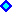Linear Models for the NPV EquationNonlinear Models for the NPV Equation

OBS: Because I use the tag "Font Symbol" for the "Greeks" letters, it is recommendable to use the browser Internet Explorer or Netscape until version 4.x. Unfortunately, Netscape versions 6.x and 7.x don't support "Font Symbol" for the "Greeks" letters anymore (I think this is a big drawback for the new versions of Netscape - a negative evolution).
If you is looking the letter "s" as "s" instead of the Greek letter "sigma", your browser doesn't support "Font Symbol".

This recommendation is also valid for many pages in this website that work with equations.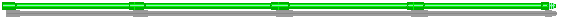## Linear Models for the NPV Equation

### 1) Introduction to Linear Models for the NPV Equation

Initially, consider only the optimal timing to exercise the option to develop a project. The payoff from exercising the option to develop the project, is the net present value (NPV). The NPV is equal to the present value of revenues net of operational costs and taxes (V) less the present value of the investment cost I net of fiscal benefits (NPV = V - I). For while, we are not including operational options, that is, options after the development (option to expand, option to stop temporally, option to abandon).

In some cases, we can adopt a simple proceeding such as the one suggested by Copeland & Antikarov's textbook (2001), considering only a geometric Brownian motion for V with volatility estimated from the distribution of present values of V at t = 1. This distribution results from a combination of uncertainties using a Monte Carlo simulation.
However, in some cases this procedure is not adequate because we want to make explicit some key variables along the model. One example is the case of value of information, where nature of the process of information revelation of technical uncertainties is totally different of a Brownian motion, so that it is not possible to combine these uncertainties to get a "total volatility" for V.
Specially for these richer cases, we need to work with payoff (NPV) functions making explicit some key variables like the price of product P, the reserve volume B, the reserve quality q, the operational costs OC, etc.

In these more complex problems we need to work with Monte Carlo simulation to combine technical and economic uncertainties. A practical challenge in petroleum projects is how to model the NPV function when performing a Monte Carlo simulation of the key factors with technical and economic uncertainties. There are at least three alternatives to consider both technical and economic uncertainties into the NPV function.
First using a model as simple as possible but considering the main uncertainties, which are parameterized from the DCF (discounted cash flow) model or drawn from a business vision of the project. This kind of model and its variations will be detailed later.
The second alternative is by working directly with the cash-flows, for example an integral with revenues and costs explicitly written as function of variables with uncertainty . This can be done also by putting formulas and correlation among cells in the spreadsheet linked to the sources of uncertainties, because the Monte Carlo simulation needs to change every cell in the appropriated way. Although this is possible, the formulas can be complex to link the uncertainties on the reserve size and productivity of wells, complicating the interpretation and with a much higher computational cost than the first alternative. This second way was used in the PUC-Petrobras research project to model an option to expand the production through new wells. This case was easier than the general case because the technical parameter was set at well level outcome.
The third alternative for a Monte Carlo simulation of the NPV function is by using more complex models and tools in tandem. The technical uncertainties are introduced into the reservoir simulator software, generating the distribution of production profile with its associated values for V and D (and so the NPV = V - D) in the NPV spreadsheet. The problem is that the reservoir simulator is called for every sample used in the Monte Carlo simulation, and the reservoir simulator (that solves a system of partial differential equations) is not fast enough, so that the computation is very slow. In the future, it will become the preferable one because uses the revelation distributions in a more realistic way.

Since the NPV is just a linear function of the expected cash-flows, it is natural to consider models which the NPV is a linear function of the price of the product (e.g., oil price P), because cash-flows in general are also linear function of P.
Linear models for the variation of V (or the NPV) with the output prices P are the most important models inside and outside the petroleum industry, due to its practical relevance.

In petroleum projects, for the fiscal regime of concessions (USA, UK, Brazil, and others), the linear equation for the NPV with the oil prices is at least a very good approximation. For the fiscal regime of production sharing (used for example in Africa), the same is not true ( see nonlinear NPV topic).

If the underlying asset V is a linear function of P, that is, V = a P + b, and if the constant a > 0, we can say that V and P are perfectly positively correlated (coefficient of correlation between V and P is equal + 1). See the proof for example in the DeGroot & Schervish's text, p.218 (Probability and Statistics, Addison-Wesley, 3rd Edition, 2002).

The development below uses a petroleum development project as guide. However, similar reasoning applies in many other industries.
Assume that D = investment cost to develop the petroleum project with volume of B barrels of reserve. This project has a random benefit V. This benefit in this petroleum case is the value of the developed reserve. A factor q or q' (depending of the model) will be named economic quality of the reserve for the petroleum case (because as higher is q, as higher is the developed reserve value V, for details on q click here).

The figure below presents two different linear equations for the NPV equation (NPV = V - D), using different definitions for the value of the underlying asset (the reserve value V). One is named "Business Model" and the other one is named "Rigid Cash Flow Model".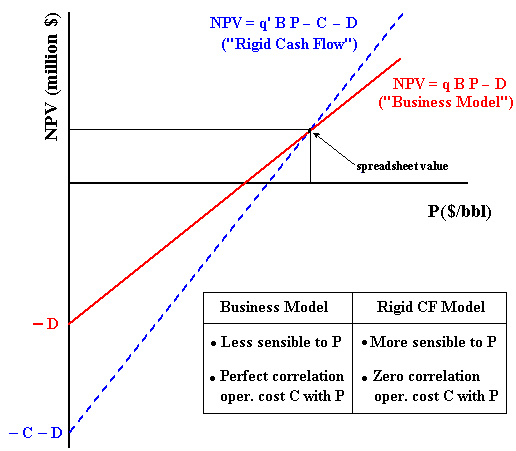In comparison with the "Business Model", the model named "Rigid Cash Flow" is more sensible to the oil prices. The intuition says that this means higher real options value F(P) for the "Rigid Cash Flow Model". In this aspect the "Business Model" is more conservative about the value of the investment timing option. This intuition will be confirmed with the numerical results of an example drawn from the Timing (Excel spreadsheet) versions for both models.
In addition, for lower oil prices the option to shut-down could have a non-negligible value (reducing the losses predicted in the "Rigid Cash Flow Model" chart).

The "Business Model" assumes that the operational cost is proportional to the price P, so that there is a perfect positive correlation between operational cost and price. The "Rigid Cash Flow Model" assumes zero correlation between the operational cost (C) and the oil price (P). In reality, microeconomics logic points that a positive correlation is expected to occur between C and P because the main costs are industry-specific. For example, wells maintenance are done by specific rigs, oil transport demands tankers, and many others petroleum services are industry-specific. The microeconomics logic of correlation means that higher oil prices tends to increase the demand for specific petroleum services, increasing the operational cost C, and vice versa.
The truth on the correlation lies between the "Business Model" (perfect positive correlation) and the "Rigid Cash Flow Model" (zero correlation). However, an intermediate model means to assume the operational cost C as stochastic, introducing some additional complexity to the model.

In the next sub-topics both models are detailed and some results and proofs are presented. Return to figure above when necessary to understand better the models.

In order to understand the "Business Model", think about the market value of one barrel of developed reserve v (that is, v is the price of the barrel of developed reserve). If this reserve price v is directly related with the long-run oil prices, let be q the factor of proportionality so that v = q P. For developed reserve transactions, as higher is the price per barrel of a specific reserve, as higher is the economic quality for that reserve. For a fixed reserve volume and fixed oil price, as higher is the factor q as higher is the value of this reserve.
By using this insight, the value of a reserve V is the price of the barrel of reserve v times the size of this reserve B, that is, V = v B. The equation for the developed reserve value V is:

V = q B P.

This is the easiest way to work with the three most relevant variables to access the value of a developed reserve, using business thinking, which is very adequate for market valuation. The value q can be assessed either by market transactions in markets like USA (see Adelman & Koehn & Silva, 1989; and Adelman & Watkins, 1996) or by using the discounted cash flow approach.
In the first case, is necessary to consider the appropriate discounting effect on q in order to put in present value the reserve value V. Note that q is the only parameter to consider the discounting effect.
In the second case, consider the spreadsheet NPV estimate for a certain (average) oil price. In a chart NPV x P this base-case NPV estimative, is one point of our straight line, the other one is defined by NPV(P=0) = - D. The figure above presents the link between this chart and the equation for the "Business Model".

The Business Model was used in the classical real options model of Paddock & Siegel & Smith (1988) (see also Dixit & Pindyck, 1994, chapter 12), where the authors assumed the one-third rule of thumb for q, that is, q = 1/3 = 33%. This is considered a mean value for the developed reserves in the United States.

#### Proof that V Follows the Same Geometric Brownian Motion of P

Let us prove that if the oil prices (P) follow a geometric Brownian motion (GBM) and the value of the project (V) is proportional to the oil prices, then V also follows a GBM and with the same parameters of P.

Consider the following risk-neutral GBM for the oil prices and the equation for V from the "Business Model":

dP = (r - d) P dt + s P dz

V = q B P

By applying the Itô's Lemma (see Dixit & Pindyck, 1994, p.80) to V(P, t):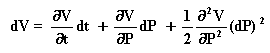However,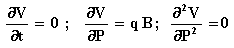Hence,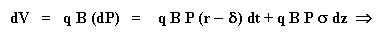Finally, we get a very familiar risk-neutral equation for the stochastic process of V: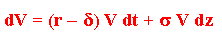This equation is the risk-neutral geometric Brownian motion for V. Even more interesting is that the parameters s and d for the stochastic processes of P and V are the same! This is one practical attractive issue that occurs with the "Business Model" but not with the "Rigid Cash Flow Model" (as we will see).

#### The Partial Differential Equation for the Real Option in the Business Model

The partial differential equation (PDE) for the real option F(P, t) in the "Business Model" is exactly the same PDE for the "Rigid Cash Flow Model"!
The differences between the two models appear in the payoff specified in the boundary conditions. The deduction of the PDE is identical to the one presented in the topic on the "Rigid Cash Flow Model".

The PDE and the 4 boundary conditions for the "Business Model" are presented below (the subscripts denote partial derivatives):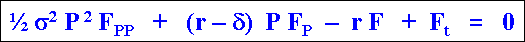1) for P = 0, F(0, t) = 0

2) for t = T (expiration), F(P, T) = max (q B P - D, 0)

3) Value matching: for P = P* (where P* = threshold for optimal immediate investment), F(P*, t) = q B P* - D

4) Smooth pasting: for P = P*, FP(P*, t) = q B

This equation can be solved with numerical methods or using the very good analytical approximation of Bjerksund and Stensland ("Closed-Form Approximation of American Options", Scandinavian Journal of Management, vol.9, 1993, pp.87-99). This approximation is used in the fast spreadsheet Timing and in the versions for both linear models presented in this webpage.

The Excel spreadsheet below, shareware available to download, is the Timing Version Business Model, which calculates real options, threshold, probability of exercise, and expected first-hitting time conditional to exercise, using the "Business Model".Download the Excel spreadsheet Timing Version Business Model (timing-business_model-vba-hqr.xls), with 702 KBOr download the compressed (.zip) version of this spreadsheet Timing Version Business Model (timing-business_model-vba-hqr.zip), with 636 KB

The only limitation of this spreadsheet compared with the registered version, is that some inputs are fixed in this non-registered version. The fixed inputs are: Initial oil price; investment cost; risk-free interest rate; and dividend yield (or convenience yield). Registered users can freely change any input.

The table below presents results from a numerical example of a real options with two years to expiration (for the default values in the Timing spreadsheets for both models), with the same values for the producing project value (V), investment (I) and Net Present Value (NPV).
This table shows that the Business Model is more conservative in terms of real option value. However, it is less conservative in terms of the option to invest exercise because it recommends earlier exercise than the Rigid Cash Flow Model.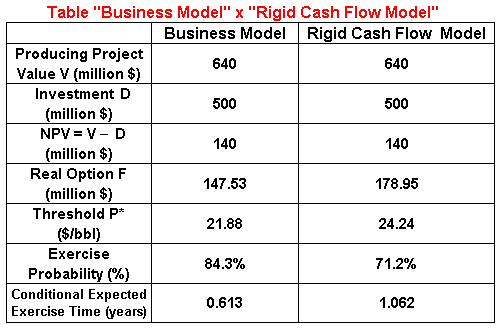Although applicable also to the Rigid Cash Flow Model, the concept of economic quality of reserve was first thought for the Business Model because it is the multiplicative factor on the oil prices in order to get the value of one barrel of developed reserve.
So, for more details on the Business Model and its applications, see the webpage on economic quality of reserve.

### 3) The Rigid Cash Flow Model

The equation for the producing project value V(P) in the "Rigid Cash Flow Model" is given by the equation:

V = q' B P - C

Where q' is the economic quality of this reserve (because it is the partial derivative of V in relation to P, per barrel). The reserve volume is denoted by B, and C is a present value of part of the operational costs. The remaining part of the operational cost (like royalties) is embedded in q', as we will see later.

The net present value (NPV) for this model is:

NPV = q' B P - C - D

There are similar models in the real options literature. For example:

• The paper of Bjerksund & Ekern (1990, see eq.10, their NPV equation). The differences are that the authors assume that all present value of the costs are embedded in the same term K. In reality it is really possible to joint the costs C and D by making D' = D + C if these parameters are constant to solve the real options problem (it will be proved later). However, I think that they exaggerated a little bit when jointing all the costs because there are some important operational costs that are function of the revenue (and so of the stochastic variable P), that in our case is embedded in q'. These operational costs include royalties over the gross revenue and income-tax and other taxes over the net revenue. However, this paper brings the useful idea to make NPV = V'(P) - D', which will be developed here later.
.
• In the textbook of Dixit & Pindyck (1994, chapter 6, section 2, eq.11). The differences are that they considered the option to temporary suspension (or option to shut down, see this nonlinear NPV topic) and considered a infinite lived project.
.
• The paper of Schwartz (1997, see eq.49, his NPV equation). As in Bjerksund & Ekern (1990), he doesn't associate any operational cost parcel to the oil price level (my main criticism on both papers equations), but he separates the operational cost from the investment.

Let us discuss a typical discounted cash flow analysis for the NPV. In order to keep things simple, assume that the discount rate r is the same for all project flows (revenues and costs) after the option exercise (a similar reasoning is possible using different discount rates).
By grouping the cash flows using our equation NPV = V - D, where V is the presented value of the revenues net of operational costs and taxes, and D is the present value of the investment net of fiscal benefits associated to the investment (net of depreciation and others fiscal benefits). The equation for V deserves more details.
In this way, we can write the following equation for V: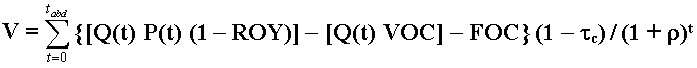Where Q(t) is the production at the year t; P(t) is the oil price; ROY is the royalties rate (generally between 10 - 15%); VOC is the variable operational cost (chemical products, transport costs, etc.); FOC is the fixed operational cost (maintenance cost in the wells and processing plant, wage costs with the operators, etc.); tc is the corporate income tax rate; r is the discount rate; and tabd is a (fixed) abandonment year.

Note in the equation above that the first term inside [.] is the revenue net of royalties, the second one is the variable operational cost, and the third one is the fixed operational cost. We can separate the above equation into three terms as follows: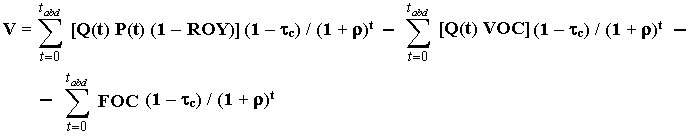For simplicity, in addition we assume that the (stochastic) long-run price P in the above equation is constant (after the option exercise) or P represents a weighted average price (first years are much more important due to both Q(t) profile and discounting effect). Of course is possible to use the curve E[P(t)] to calculate the first term, but it costs computational time and loses analytical facilities (e.g., it is not possible to use the analytical approximation for American calls options).
In this way we can isolate P and other terms which are not function of the time in the equation above, writing: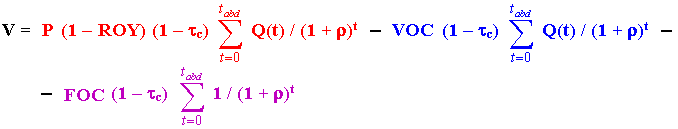Comparing the above equation with the "Rigid Cash Flow Model" equation for V(P) = q' B P - C, the first term (in red) corresponds to q' B P, and the summation of the remaining terms (in blue and in violet) corresponds to C (the terms without P). The term in blue corresponds to the variable operational costs, whereas the term in violet are the fixed operational costs.

This means that, in the Rigid Cash Flow Model, the quality factor q' is a function of the royalties, the income tax rate, and the discount rate. In this case the Economic Quality of a Reserve q' for the Rigid Cash Flow Model is given by: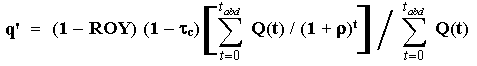Because the summation with Q(t) alone is exactly the reserve volume B. It is easy to prove that q' < 1 for positive values of the parameters of the above equation.

By looking the blue term in the last equation for V, it is obvious that the operational cost factor C is also related with the reserve volume B. What about the last term (the violet one)? Although it is not visible in the equation, it is also related with the reserve volume B. Why? Remember that the fixed operational cost is related with the capital in place, that is, it is (an increasing) function of the number of wells and the size of the production system (platform size, etc.). So, as in the case of the development investment D, the operational cost factor C is also an increasing function of the reserve size B and we can write C(B) in real options applications like the ones related with the value of information (models with technical uncertainty).

For this case, we can write the equation for the operational factor C as: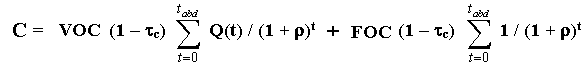In practice, the easiest way to find the factors q' and C is by performing the sensitivity analysis of the NPV with P in a discounted cash flow spreadsheet. With resulting linear equation for the NPV x P, the intercept is - C - D, whereas the angular coefficient is q' B. Return to the introduction to linear models and see the linear chart NPV x P, in order to remember this point.

#### Contingent Claims Deduction of the Partial Differential Equation for the Real Option F(P, t)

Let us relate the value of real option to invest in the project F with the stochastic variable oil price P for the "Rigid Cash Flow Model". We want the partial differential equation (PDE) for the value of real option F(P, t). This option expires at t = T and the exercise earn the payoff:
NPV = q' B P - C - D

In the contingent claims method (see Dixit & Pindyck, for example) we need to construct a riskless portfolio F relating the option F with the stochastic variable P. Assume an imaginary portfolio buying one option F and selling short n units of P (or n barrels of oil). The value of this portfolio is:

F = F - n P

The value of n will be conveniently chosen in order to make riskless this portfolio (delta hedge). In this way, the riskless return of this portfolio in a small interval dt is given by:

r F dt = r ( F - n P ) dt

But the portfolio return is also the algebraic sum of the return from its individual components.
The variable F return is related only to capital gain (it doesn't pay dividends). This capital gain in a small interval dt is denoted by dF.
The return of the variable P in a small interval dt has two components, the capital gain dP and the dividend d P dt, where in d is the convenience yield of this commodity (or rate of return of shortfall). So, the portfolio return is also:

r F dt = dF - n (dP + d P dt)

Equaling the two equations:

r ( F - n P ) dt = dF - n (dP + d P dt) . . . . . . . (*)

The value of dF is given by the Itô's Lemma expansion for F(P, t):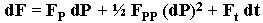Where the subscripts denote partial derivatives. If P follows a geometric Brownian motion (GBM), elementary stochastic calculus tells us that (dP)2 = s2 P2 dt, we have: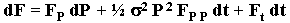Substituting dF into the returns equation (*), we obtain: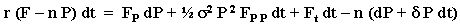Rearranging, we get: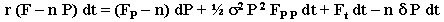We want that the portfolio return, given by the equation above, be riskless. This means that we need to eliminate the stochastic term with dP (which is function of dz) to get a riskless return. This is easily performed by choosing the value of n = FP in the above equation. So, we obtain: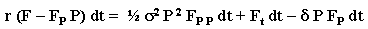Rearranging we finally get the PDE for the real option F in function of the stochastic variable P:There are interesting coincidences with the above PDE. First, in financial options, if P is the price of a stock, the above PDE is the exactly the famous Black-Scholes-Merton equation (version with continuous dividends)! Second, the reader can verify that the PDE for the "Business Model" is exactly the same above!
Why? This PDE only relates the option value F with P. Note that until this point, the payoff equation that distinguishes the "Rigid Cash Flow Model" from the "Business Model" was not used yet. As in many options models cases (but not all), the differences are in the boundary conditions:

a) for P = 0, F(0, t) = 0

b) for t = T (expiration), F(P, T) = max (q' B P - C - D, 0)

c) Value matching: for P = P* (where P* = threshold for optimal immediate investment),
F(P*, t) = q' B P* - C - D

d) Smooth pasting: for P = P*, FP(P*, t) = q' B

However, if both the operational cost C and the development investment D are constants (given the expected values for q' and B), we can solve the "Rigid Cash Flow Model" using the software that solves the "Business Model"!
How? Look the differences, the boundary conditions. If we call V' = q' B P = V + C and also D' = D + C, we can use for example our Excel spreadsheet Timing to solve the "Rigid Cash Flow Model" (available to download below).

Using V' and D', we can use even the concept of homogeneity of degree 1 described in Dixit & Pindyck (p.210) because V' is proportional to P. For the case of costs (both C and D) this property helps to solve many problems (like the one related to value of information with constant values for the cost C and investment D). This is because we can use a normalized threshold curve [V'/D'(t)]* = [(q' B P*(t))/(D + C)] to fasten the problem solution.

However, when including stochastic costs the situation is very different. In order to hold the degree 1 homogeneity is necessary to assume that D' (= C + D) evolves according a (correlated with P) geometric Brownian motion. The concept of homogeneity will not apply to D itself (imagining either C constant or following other stochastic process). The intuition behind is simple. If we double P and D but not C, the NPV will not be the double, and so the option for the cases when F = NPV. However, if P, C and D are doubled, the NPV will be the double.
The harder to accept assumption that C + D follows a GBM (in order to take advantage of the homogeneity) is the main disadvantage of the "Rigid Cash Flow Model" compared with the "Business Model".

For the case when P, C and D follow different (correlated) stochastic processes, even GBMs, the real options PDE, F(P, C, D, t) can be complicated to solve by finite differences. In this case, recent techniques of Monte Carlo simulation for American options looks the best practical way to solve this problem.

The Excel spreadsheet below, shareware available to download, is the Timing Version Rigid Cash Flow Model, which calculates real options, threshold, probability of exercise, and expected first-hitting time conditional to exercise, using the "Rigid Cash Flow Model". It considers that the (long run expectation on) oil prices follows a geometric Brownian motion (GBM).Download the Excel spreadsheet Timing Version Rigid Cash Flow Model (timing-rcf_model-vba-hqr.xls), with 703 KBOr download the compressed (.zip) version of this spreadsheet Timing Version Rigid Cash Flow Model (timing-rcf_model-vba-hqr.zip), with 637 KB

The only limitation of this spreadsheet compared with the registered version, is that some inputs are fixed in this non-registered version. The fixed inputs are: Initial oil price; investment cost; operational cost factor; risk-free interest rate; and dividend yield (or convenience yield). Registered users can freely change any input.

Again, the table below presents results from a numerical example of a real options with two years to expiration (for the default values in the Timing spreadsheets for both models), with the same values for the producing project value (V), investment (I) and Net Present Value (NPV).
This table shows that the Rigid Cash Flow Model is less conservative in terms of real option value. However, it is more conservative in terms of the option to invest exercise because it recommends later exercise than the Rigid Cash Flow Model.## Nonlinear Models for the NPV Equation

### 1) Fiscal Regime of Production Sharing: Chart NPV x P

The chart below presents the typical sensitivity analysis NPV with the oil prices P, for the fiscal regime of production sharing. This fiscal regime is used in many countries (mainly in Africa). Remember that for the fiscal regime of concession, this chart is a straight line.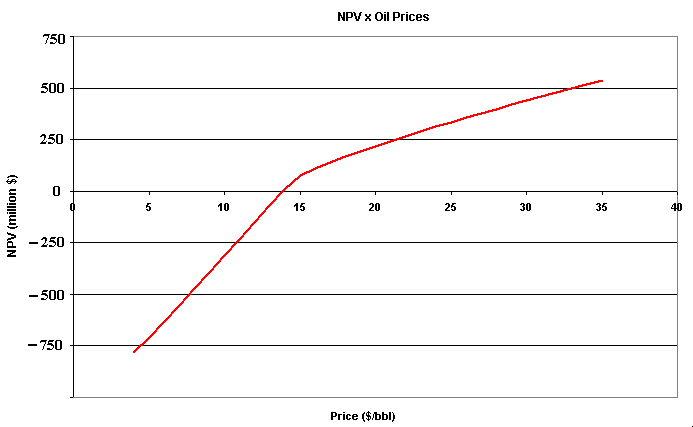The production-sharing regime has two main phases, and the figure above illustrates these two different phases. The first one is named cost recovering, so that the revenues net of operational cost from the first years are destined to the oil companies, in order to recover the amount invested in the petroleum field (in general considering an interest like Libor plus x%). In this phase, the Government Take (GT) is inexistent or very small.
The second phase, named profit phase, the revenues net of operational cost are destined to both Govern (larger part) and oil companies. It seems like two different regimes, if the project (ex-post) has no profit, the GT is zero or very small, whereas for ex-post profitable projects the GT is significant.
Remember that for the regime of concessions, profit or non-profit project is an oil company problem. Even with negative NPV, the oil companies pay royalties, income tax (if the company is profitable, doesn't matter the project), and other taxes. In the regime of production sharing, the taxation changes at the point that the project enters in the profit phase, causing the nonlinearity.

In the figure, if the oil price is under \$ 15, the project has lower fiscal charge because it stays in the cost recovering phase, but for higher prices the fiscal charge is heavier because the project reaches the profit phase. This change of regimes is not at the NPV = 0 level because the discount rate used in the NPV estimate above is different of the discount rate used by the National Agency to reward the investment for cost recovery rule purposes.

This case is a bit more complex to simulate, but it is not so critical. It requires a complete specification of the NPV function variation with the oil prices (like the above chart) and with the others variables of interest (like reserve volume, productivity of the reserve, etc.).

### 2) Net Present Value with Option to Shut-Down

For the model developed in the book of Dixit & Pindyck, chapter 6 (p.188, eq.12), we have the value of the underlying asset V(P) considering a nonlinear term due to the (costless) option to shut-down the production if the price P drops below the unitary operational cost c. The NPV equation is equal to V(P) less the investment I.
Although the textbook cash-flow function max(P - c, 0) is too simple, the model permits to develop the intuition on the effect of the shut-down option in both the NPV and the option to invest. This intuition generally holds in more complex cases.

The chart below presents an example of the NPV equation with the option to shut-down.: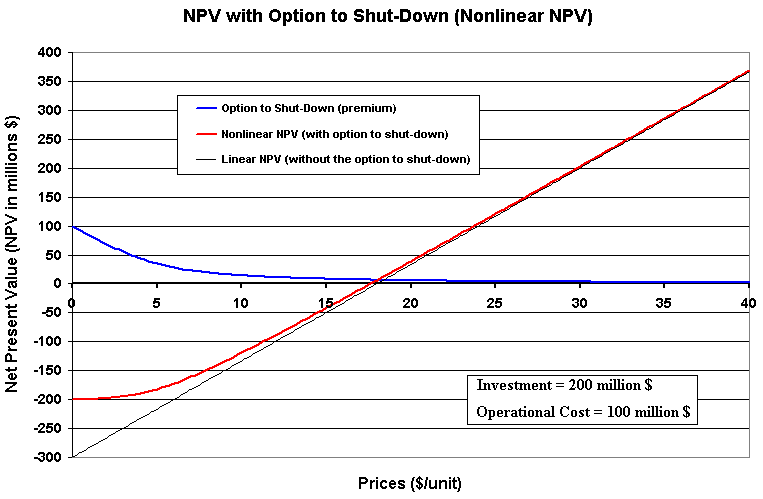The case in the book refers to a project with infinite production life. This assumption permits an analytical solution for the NPV with the (costless) option to shut-down. For projects with finite life, including the option to shut-down will demand a numerical solution for the partial differential equation that determines the value of the underlying asset V(P, T), and so the NPV = V - I.

In addition, it permits to calculate the value of a perpetual option to invest in this project (the option to wait and see, see Dixit & Pindyck p.190), generating also the chart for the perpetual timing option presented below.
In this case the payoff from the exercise of the option to invest in the project, is the NPV of the project including an option to shut-down (this spreadsheet doesn't use macros or VBA and is not password protected).Download the Excel spreadsheet dp-chapter6-nonlinear_npv.xls, with 108 KB

This spreadsheet also illustrates the application of the Newton-Raphson method to solve nonlinear equations. In the above spreadsheet, this method gets automatically the value of the threshold P* and consequently the value of the real option F.

The chart of the perpetual option to invest with the price P is displayed below.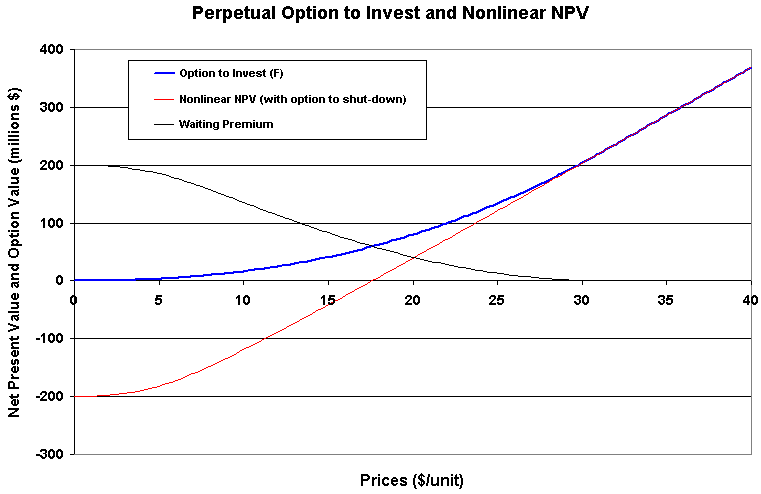Back to the Petroleum Models Menu

Back to Contents